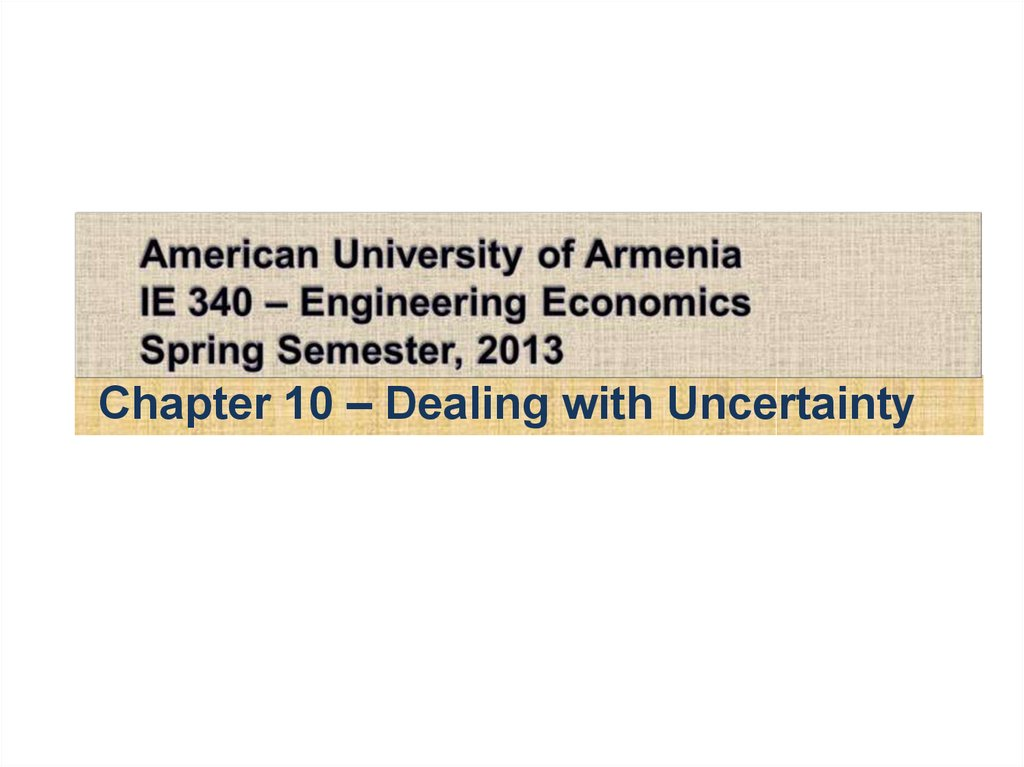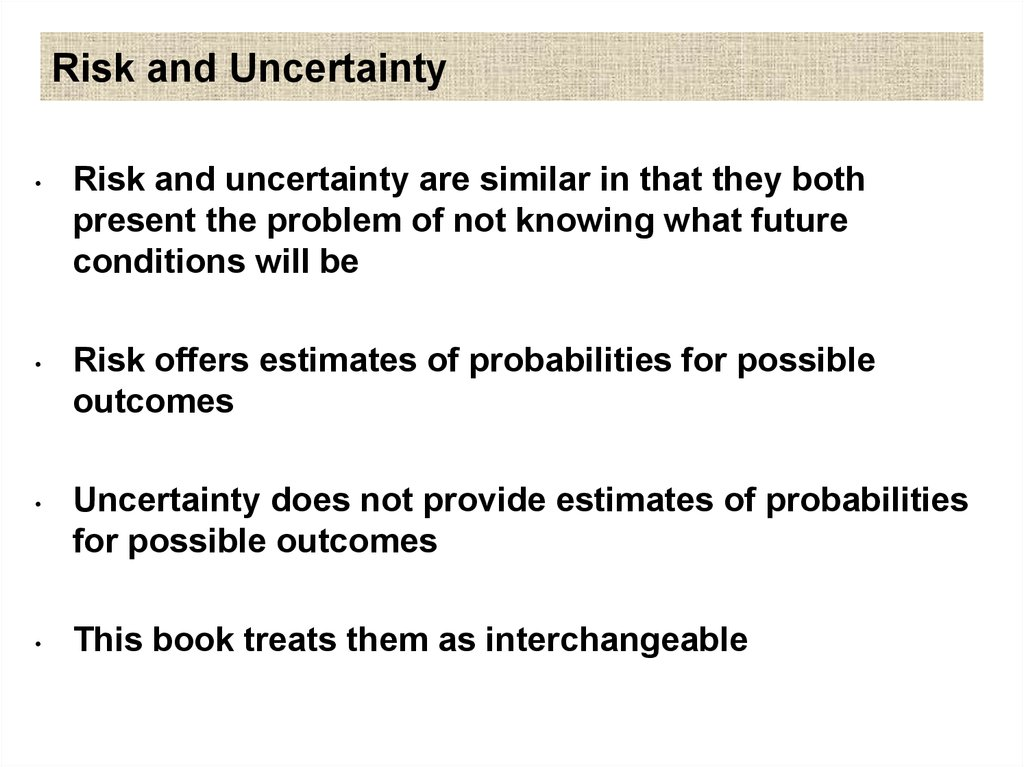# Risk and Uncertainty

## 1.

Chapter 10 – Dealing with Uncertainty

## 2. Risk and Uncertainty

Risk and uncertainty are similar in that they both
present the problem of not knowing what future
conditions will be
Risk offers estimates of probabilities for possible
outcomes
Uncertainty does not provide estimates of probabilities
for possible outcomes
This book treats them as interchangeable

## 3. Four major Sources of Uncertainty

1.
Possible inaccuracy of cash-flow estimates used in
the study
2.
Type of business relative to the future health of the
economy
3.
Type of physical plant and equipment involved
4.
Length of study period

## 4. Possible Inaccuracy of Cash-flow estimates

How much source information is available
How dependable is the source information
Uncertainty
in
capital
investment
requirements is often reflected as a
contingency above actual cost of plant and
equipment

## 5. Type of Business Involved Relative to Health of Economy

Some businesses will typically be more at risk
of declining with when there is a general
decline in the economy -- when the economy
has gone into recession

## 6. Type of Physical Plant and Equipment Involved

Some types of structures and equipment have definite
economic lives and market values – they may be used
in a multitude of settings
Other dwellings and equipment, being made for very
specific and singular functions, may have little or no
resale value

## 7. Length of Study Period

The longer the study period, the greater the
level of uncertainty of a capital investment

## 8. Sensitivity Analysis

Sensitivity – The degree to which a
measure of merit (i.e., PW, IRR, etc…) will
change as a result of changes in one or
more of the study factor values.
Sensitivity Analysis Techniques
1.
Breakeven Analysis
2.
Sensitivity Graph (spider-plot)
3.
Combination of factors

## 9. Breakeven Analysis

Technique commonly used when an uncertain single
factor (EG: capacity utilization) determines the
selection of an alternative or acceptability of an
engineering project
For given alternative, if best estimate of actual
outcome of common factor is higher or lower than
the breakeven point, and assumed certain, the best
alternative becomes apparent

## 10. Breakeven Analysis

Indifference between alternatives
(EWA = f1(y); EWB = f2(y)
EWA = EWB; f1(y) = f2(y) : Solve for y
Economic acceptability of engineering project
EWp = f(z) = 0
The value of ‘z’ is the value at which we would
be indifferent between accepting or rejecting
the project

## 11. Breakeven Problem Involving Two Alternatives

Most easily approached mathematically by
equating an equivalent worth of the two
alternatives expressed as a function of the
factor of interest

## 12. Breakeven Analysis for Economic Acceptability of an Engineering Project

Most easily approached by equating an
equivalent worth of the project to zero as a
function of the factor of concern
Because of the potential difference in project
lives, care should be taken to determine
whether the co-terminated or the
repeatability assumption best fits the
situation

## 13. Example applications of Breakeven Analysis

Annual revenue and expenses
Rate of return
Market (or salvage) value
Equipment Life
Capacity utilization

## 14. Example

• Two electric motors are being considered to
power an industrial hoist. Each is capable of
providing 90 hp. Pertinent data for each motor
are presented bellow.
• If the expected usage of the hoist is 500 hr per
year, what would the cost of electrical energy
have to be (in cents per kilowatt-hour) before the
D-R motor is favored over the Westhouse
motor? The MARR is 12% per year. [Note: 1hp =
0.746KW]

## 15. Example

Motor
D-R
Capital investment
Westhouse
\$2,500
\$3,200
Electrical ef ciency
0.74
0.89
Maintenance per
\$40
\$60
10 yr
10 yr
year
Useful life

## 16. Example: Solution

• Let X = electrical energy cost in \$/kW-hr. Equate the equivalent
uniform annual worth of both motors:
AWD-R(12%) = AWWH(12%)
• -\$2,500(A/P,12%,10) - \$40 - (90 hp/0.74)(0.746 kW/hp)(500 hrs)(X /
kW-hr)
• = -\$3,200(A/P,12%,10) - \$60 - (90 hp/0.89)(0.746 kW/hp)(500 hrs)(X
/ kW-hr)
• \$482.5 + (\$45,364.87)(X) = \$626.4 + (\$37,719.10)(X)
• X = \$143.90 / \$7,645.77 = \$0.0188 / kW-hr or 1.88¢ / kW-hr

## 17. Sensitivity Grapfh (Spider-plot)

An analysis tool applicable when the
breakeven analysis does not fit the project
situation
Makes explicit the impact of uncertainty in
the estimates of each factor of concern on
the economic measure of merit

## 18. EXAMPLE 10-4

• The best cash-flow estimates for a
machine being considered for installation:
• Capital Investment (I) = \$11,500
• Revenues/yr (A)
= \$5,000
• Expenses
(A)
= \$2,000
• Market Value(MV)
= \$1,000
• Useful Life (N)
= 6 years

## 19. EXAMPLE 10-4

Investigate PW over a range of + 40% changes in
estimates for
a. Capital investment
b. Annual net cash flow
c. Market value
d. Useful Life
PW(10%) = -\$11,500 + \$3,000 (P / A, 10%, 6) +
\$1,000 (P / F,10%, 6) = \$2,130

## 20. EXAMPLE 10-4

• (a) Capital investment varies by + - p
PW(10%) = -(1+_ p%/100)*\$11,500 + \$3,000(P/A, 10%, 6) +
\$1,000(P/F, 10%, 6)
• (b) Annual cash flow varies by + - a
PW(10%) = - \$11,500 +
(1+_ a%/100)*\$3,000(P/A, 10%, 6) + \$1,000(P/F, 10%, 6)
• (c) Market Value varies by + - s
PW(10%) = - \$11,500 +
\$3,000(P/A, 10%, 6) + (1+_ s%/100)*\$1,000(P/F, 10%, 6)
• (d) Useful life varies by + - n
PW(10%) = - \$11,500 +
\$3,000(P/A, 10%, (6+_n%/100)) + \$1,000(P/F, 10%, (6+_n%/100))

PW (10%)
7000
6000
5000
\$2130
4000
3000
2000
-% Deviation
Changes in
Factor
Estimate
1000
- 40 -30 -20 -10
0
-1000
-2000
-3000
-4000
+10 +20 +30 +40
+%Deviation
Changes in
Factor
Estimate

## 22. Revelations of Spider-plot

Shows the sensitivity of the present worth to percent
deviation changes in each factor’s best estimate
Other factors are assumed to remain at their best estimate
values
The relative degree of sensitivity of the present worth to
each factor is indicated by the slope of the curves (the
“steeper” the slope of a curve the more sensitive the present
worth is to the factor)
The intersection of each curve with the abscissa shows the
percent change in each factor’s best estimate at which the
present worth is zero

## 23. Revelations of spider-plot

In this example
Present worth is insensitive to MV
Present worth is sensitive to I, A, and N

## 24. Measuring Sensitivity by a Combination of Factors

1.
Develop a sensitivity graph for the project
a. For most sensitive factors, improve
estimates and reduce range of uncertainty
2.
Use sensitivity graph to select most sensitive
project factors. Analyze combined effects of
these factors on project’s economic measure of
merit by:
a. Additional graphical technique for two most
sensitive factors
b. Determine the impact of selected
combinations of three or more factors -scenarios

## 25. Pitfalls of Risk Adjusted MARR

A widely used industrial practice for including
some consideration of uncertainty is to increase
the MARR
Even though intent of risk-adjusted MARR is to
make more uncertain projects appear less
economically attractive, opposite may appear to
be true
Cost-only projects are made to appear more
desirable as the interest rate is adjusted upward to
account for uncertainty

## 26. Reduction of Useful Life

By dropping from consideration those revenues
(savings) and expenses that may occur after a
reduced study period, heavy emphasis is placed
on rapid recovery of capital in early years of a
project’s life
This method is closely related to the discounted
payback technique and suffers from most of the
same deficiencies